# 详解冒泡排序

2020年3月23日15:07:21评论59阅读模式

### 基本思想

（如下图，图片来源于网络）文章源自随机的未知-https://sjdwz.com/11137.html

### 例子

[ 5 , 8 , -2 , 20 -6 ]

(1) 5 < 8不用发生交换文章源自随机的未知-https://sjdwz.com/11137.html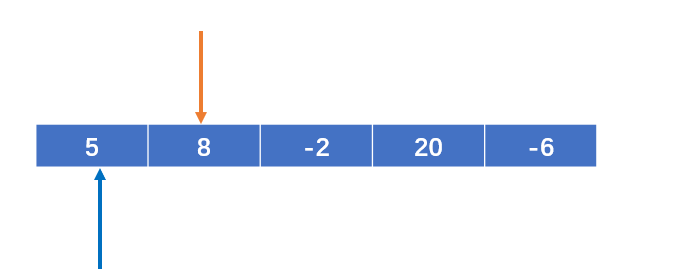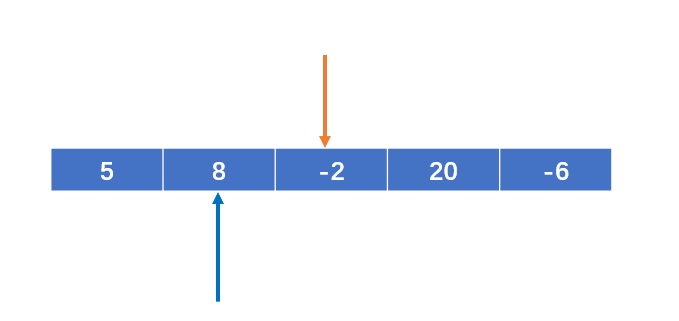(2) 8 > -2 需要发生交换文章源自随机的未知-https://sjdwz.com/11137.html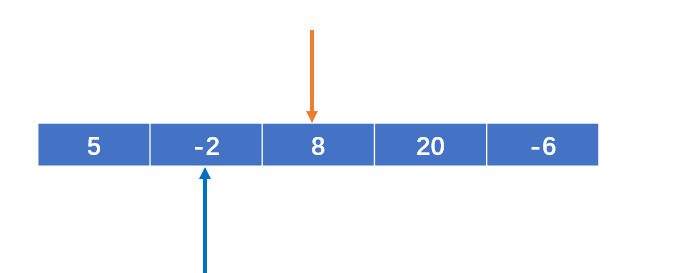(3) 8 < 20 不需要发生交换文章源自随机的未知-https://sjdwz.com/11137.html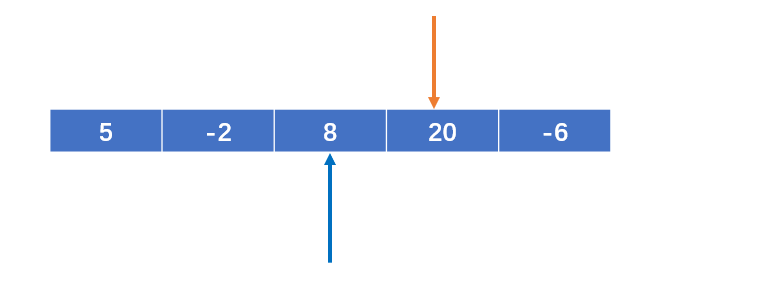(4) 20 > -6 需要发生交换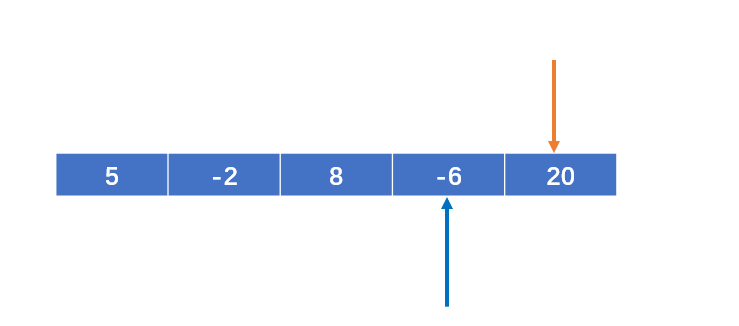文章源自随机的未知-https://sjdwz.com/11137.html

i ,j重新赋值如下：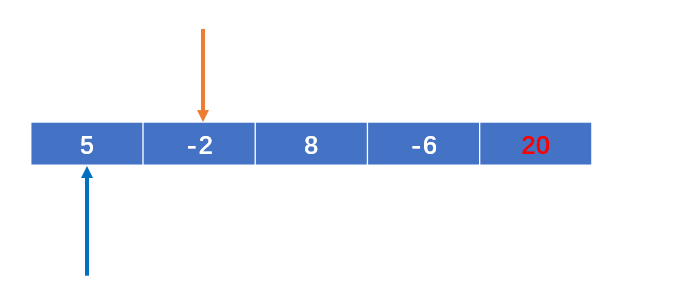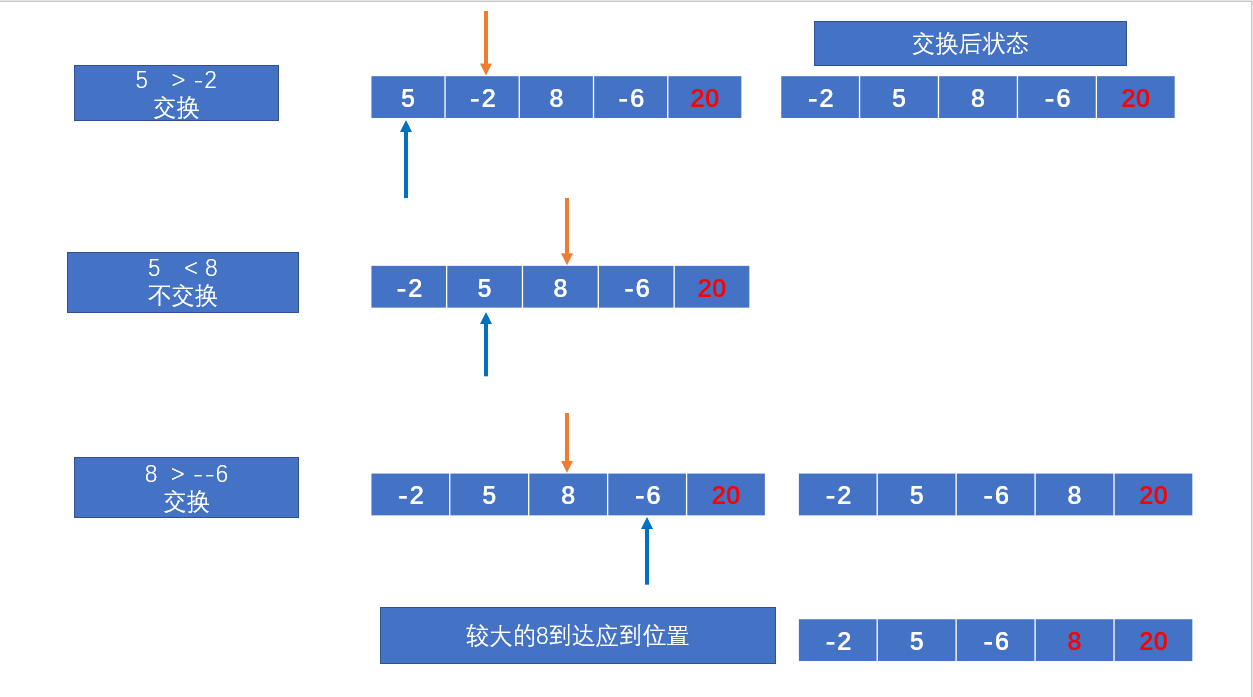文章源自随机的未知-https://sjdwz.com/11137.html

i ,j重新赋值如下：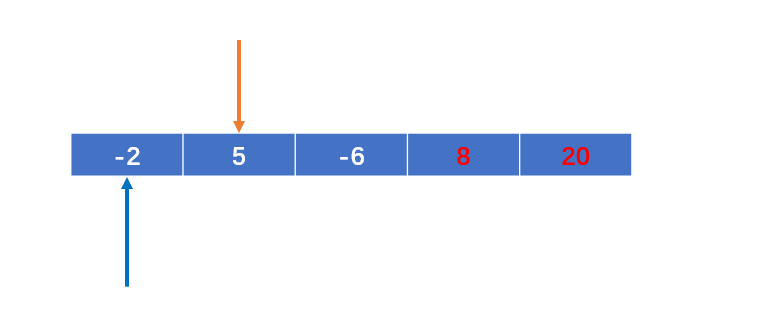文章源自随机的未知-https://sjdwz.com/11137.html

i ,j重新赋值如下：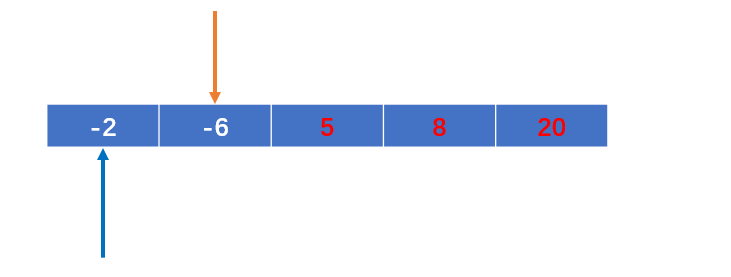文章源自随机的未知-https://sjdwz.com/11137.html

### 代码

``````int temp;//用于交换的临时变量
for(int i=0;i<arr.length - 1;i++){
for(int j = 0;j<arr.length - 1 - i ; j++){
if(arr[j] > arr[j + 1]){
temp = arr[j];
arr[j] = arr[j+1];
arr[j+1] = temp;
}
}
}
``````

### 优化

``````import java.lang.reflect.Array;
import java.util.Arrays;

public class Solution {
public static void main(String[] args) {
bubbleSort(new int[]{5,8,-2,20,-6});
}
public static void bubbleSort(int[] arr) {

int temp;//用于交换的临时变量
boolean flag = false;//表示是否进行交换
for(int i=0;i<arr.length - 1;i++){
for(int j = 0;j<arr.length - 1 - i ; j++){
if(arr[j] > arr[j + 1]){
flag = true;
temp = arr[j];
arr[j] = arr[j+1];
arr[j+1] = temp;
}
}
if(!flag){
break;
}else{
flag = false;//将flag重新置为false
}
}

System.out.println(Arrays.toString(arr));
}
}
``````

### 时间复杂度

;

n-1 + n-2 + … + 2 + 1 = = ;

### 欢迎关注文章源自随机的未知-https://sjdwz.com/11137.html

• 本文由 发表于 2020年3月23日15:07:21
• 转载请注明：来源：随机的未知 本文链接https://sjdwz.com/11137.html